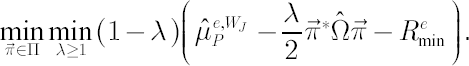The Theory \ Details \ portfolio optimization \ robust optimization

# Portfolio Optimization

## Worst-case scenario optimization

The traditional portfolio optimization framework assumes that parameters of the analytical model are known precisely. In practice, however, statistical estimates of parameters may have significant estimation error. In particular it is relevant for the sample estimateof vector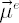that represents the excess part of expected instantaneous rates of return. Ignorance of estimation error inusually leads to unwarrantably extreme values of portfolio weights and dramatic shifts in portfolio structure when previous estimates are modified with recent historical data.

One way to avoid the mentioned above undesirable properties of an optimal portfolio is to include the estimation error explicitly in optimization process. Most practically sound results in this direction were obtained in
[Garlappi, Uppal, Wang; 2005]. The authors implement the following algorithm, which they call Multi-Prior Approach:
1. The set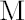of possible values for the true vectoris defined.
2. The optimal portfolio, corresponding to relative risk aversion coefficient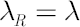, is obtained by solving the following Min-Max problem:Note. To consider the robust version of the target shortfall probability criterion the above Min-Max problem must be modified in the following way:In other words, the investor seeks for portfolio with the best performance under the worst-case scenario. Inner minimization over expected instantaneous excess returns in the above expressions can be regarded as reflection of investor’s uncertainty aversion. A convenient measure for uncertainty aversion degree is confidence level, used below to define. Asapproaches 1, investor’s aversion to uncertainty increases to infinity.

Two versions of setare considered below.

### Separate Confidence Intervals

Letbe the number of portfolio components andbe sample counterpart of. For every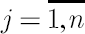corresponding confidence interval for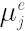in the form of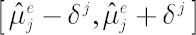is obtained by solving the following equation: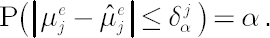The above equation has the following solution: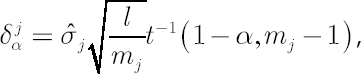where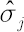– sample volatility in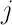-th asset– average number of observations in one year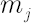– available number of observations in-th asset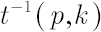– inverse function of Student’s t-distribution with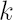degrees of freedom: it returns value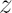such that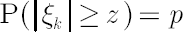, where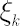is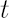-distributed random variable withdegrees of freedom.
Set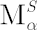, corresponding to Separate Confidence Intervals for, is defined as-dimensional rectangular parallelepiped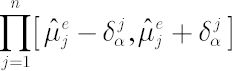.
Then, the stated Min-Max problem, corresponding to
relative risk aversion coefficient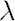, reduces towhere portfolio worst-case excess Mu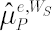is defined in the following way: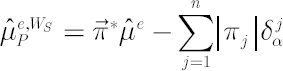Analogously, the Min-Max problem, corresponding to the target shortfall probability criterion, simplifies to following:In case of uncorrelated assets the probability of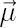falling into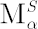is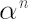. In the general case calculation of the probability of falling intois more complicated. It could be desirable to explicitly state the probability of falling intothan defining it implicitly by means of confidence intervals for individual assets. This is done in the next section.

### Joint Confidence Interval

Suppose all portfolio components possess the same number of observations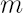. Consider the quantity, which is a sample estimate of squared instantaneous Sharpe ratio for tangency portfolio, corresponding to portfolio excess Mu equal to. This quantity is a convenient measure for the distance betweenand.

Joint Confidence Interval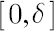for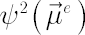is defined in the following way: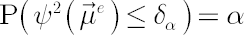Solution for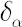has the following form: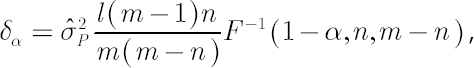where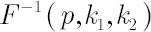is an inverse function of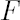-distribution withand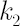degrees of freedom: it returns value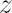such that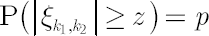, whereis-distributed random variable withanddegrees of freedom.
Set, corresponding to the above joint confidence interval for, is defined as follows: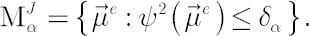The Min-Max problem, corresponding to risk aversion coefficient, simplifies to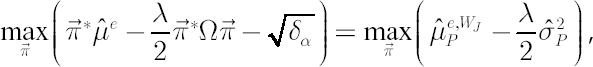where portfolio worst-case excess Mu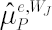is defined as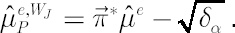Correspondingly, the Min-Max problem, corresponding to the target shortfall probability criterion, simplifies to the following: